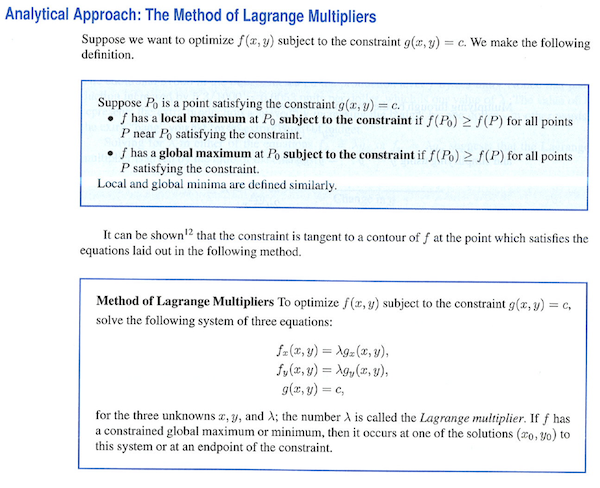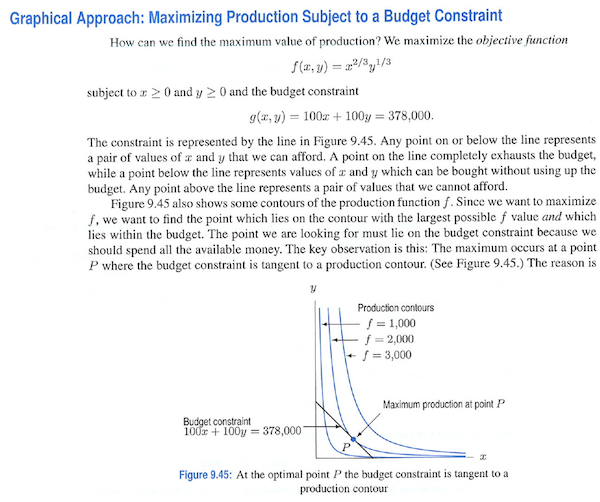# Lagrange Multipliers

Here's part of the explanation of constrained optimization from Hughes-Hallett et al. Applied Calculus 4/e (p. 383):#### QUESTION

Why does this method work?

This is an introduction to an algorithm for solving constrained optimization problems.

Before introducing an algorithm, better to introduce some context and concepts:

• Objective and constraint functions. Why do we distinguish?
• The constraint has an impact (sometimes) on the output of the objective function. What's the relationship? (Shadow prices)

Here's another explanation from the same book (p. 382).This makes it clearer why there is a tangency relationship at the optimum. But it still treats the constraint as an equation rather than as a function.